Question

A 1.000-g sample of iron metal reacted with sulfur powder to give 1.574 g of prod...

5. A 1.000-g sample of iron metal reacted with sulfur powder to give 1.574 g of prod Calculate the empirical formula of the iron sulfide.

6. A 1.000-g sample of iron metal reacted with sulfur powder to give 1.861 g of product Calculate the empirical formula of the iron sulfide.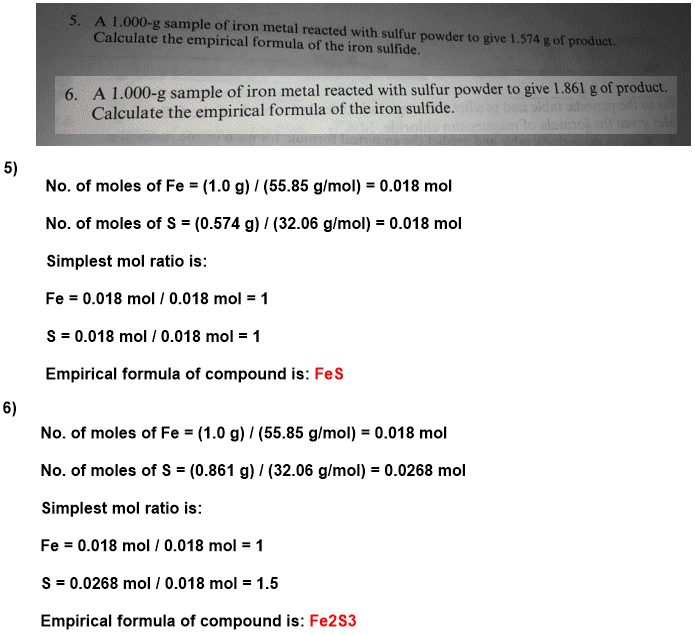Earn Coins

Coins can be redeemed for fabulous gifts.

Similar Homework Help Questions
• 5. A 0.565-8 sample of cobalt metal reacted with excess sulfur powder to give 1.027 g of cobalt sulfide

5. A 0.565-8 sample of cobalt metal reacted with excess sulfur powder to give 1.027 g of cobalt sulfide Calculate the empirical formula of the product. 6. A 0.750-g sample of tin foil was heated in air and reacted with oxygen gas to give 0.953 g of tin oxide. Calculate the empirical formula of the product. 7. (optional) A 1.000-g sample of red phosphorus powder was burned in air and reacted with oxygen gas to give 2.291 g of phosphorus oxide. Calculate...

• 1.167-g sample of iron powder reacted with 0.680 g of sulfur powder to give iton oduct....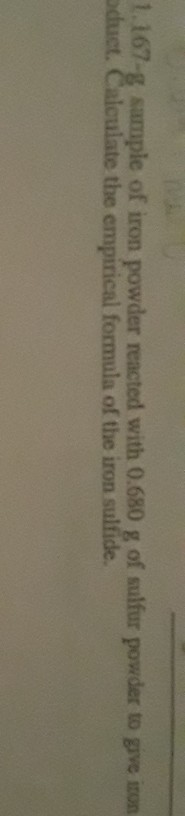1.167-g sample of iron powder reacted with 0.680 g of sulfur powder to give iton oduct. Calculate the empirical formula of the iron sulfide.

• A 0.500-g sample of chromium metal reacted with sulfur powder to give 0.963 g of product.   a) The mass of sul...

A 0.500-g sample of chromium metal reacted with sulfur powder to give 0.963 g of product.   a) The mass of sulfur that reacted is _____ b) The empirical formula of the chromium sulfide is _____

• 40.505-8 sample of cobalt metal reacted with excess sulfur powder to give 1.027 g of cobalt...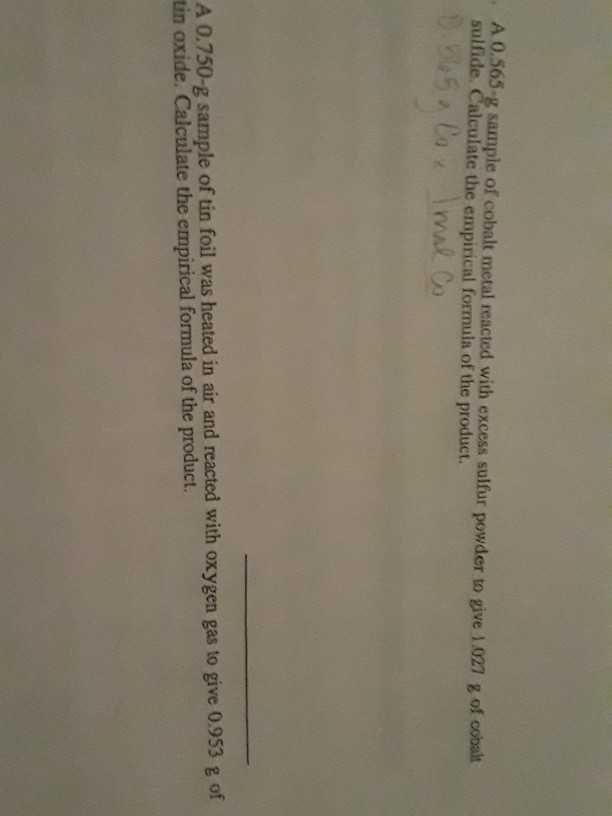40.505-8 sample of cobalt metal reacted with excess sulfur powder to give 1.027 g of cobalt sulfide. Calculate the empirical formula of the product. D. 565. Cox Imal Co A 0.750-g sample of tin foil was heated in air and reacted with oxygen gas to give 0.953 g of tin oxide. Calculate the empirical formula of the product.

• A 1.000-g sample of cobalt metal reacted with sulfur powder to give 1.548 g of product....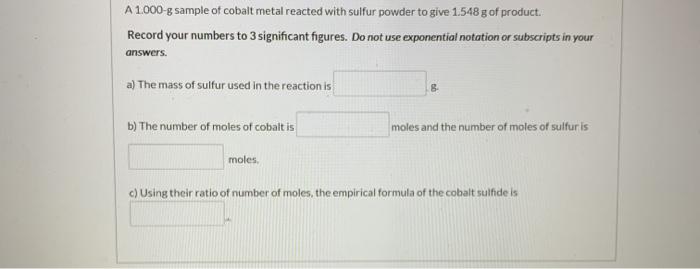A 1.000-g sample of cobalt metal reacted with sulfur powder to give 1.548 g of product. Record your numbers to 3 significant figures. Do not use exponential notation or subscripts in your answers. a) The mass of sulfur used in the reaction is b) The number of moles of cobalt is moles and the number of moles of sulfur is moles. c) Using their ratio of number of moles, the empirical formula of the cobalt sulfide is Draw the Lewis...

• A 2.41g sample of iron powder (Fe) reacted with 1.405 g of sulfur(S)

A 2.41g sample of iron powder (Fe) reacted with 1.405 g of sulfur(S). Calculate the empirical formula of the iron sulfide product.

• 5-8 sample of cobalt metal reacted with excess salfiur powder to give 1.00 Calculate the empirical...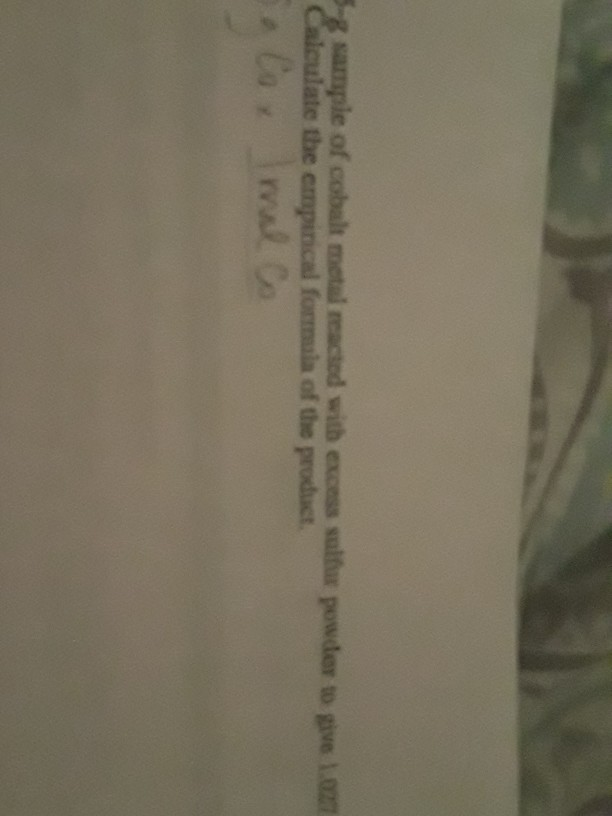5-8 sample of cobalt metal reacted with excess salfiur powder to give 1.00 Calculate the empirical formula of the product olo. Imal Co 55-g sample of cobalt metal reacted with excess sulfur powder to give 1.027 - Calculate the empirical formula of the product.

• homework

1. A 0.565 sample of cobalt metal reacted wtih excess sulfur powerd to give 1.027g of cobalt sulfide. Calculate the empirical formaula of the product.2.A. 0.750g sample of tin foil was heated in air and reacted with oxygen gas to give 0.953g of tin oxide. calculate the empirical formula of the product/3. A. 1.167g sample pf iron powder reacted with 0.680g of sulfur powder to give iron sulfide product. Calculate the empirical formula of the iron sulfide.

• -- 0 02 -.UJU U CILJI UUUU. 26. A 3.78 g sample of iron metal is...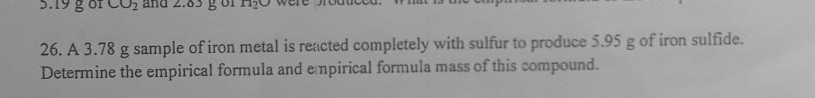-- 0 02 -.UJU U CILJI UUUU. 26. A 3.78 g sample of iron metal is reacted completely with sulfur to produce 5.95 g of iron sulfide. Determine the empirical formula and enpirical formula mass of this compound.

• 1. A 0.750-g sample of tin metal reacts with 0.201 g of oxygen gas to form tin oxide.

1. A 0.750-g sample of tin metal reacts with 0.201 g of oxygen gas to form tin oxide. Calculate the empirical formula of the tin oxide. 2. A 0.565-g sample of cobalt metal reacts with excess sulfur to give 1.027 g of cobalt sulfide. Calculate the empirical formula of the product. 3. A 1.164-g sample of iron filings reacts with chlorine gas to give 3.384 g of iron chloride. Calculate the empirical formula of the product? 4. A 0.626-8 sample of copper oxide...